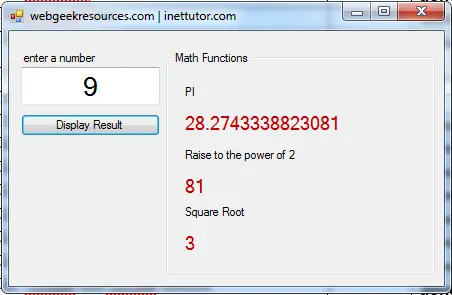# Math Functions in Visual Basic .Net• Version
• File Size 159.66 KB
• File Count 1
• Create Date April 6, 2016
• Last Updated April 6, 2016

# Math Functions in Visual Basic .Net

Math Functions in Visual Basic .Net
Math function included in this sample vb.net program are the following; pi, square root, and power of 2.

The program will let you enter a number then the program will get its square root and raise that number to the power of 2.

Source code:

```Public Class Form1

Private Sub Button1_Click(ByVal sender As System.Object, ByVal e As System.EventArgs) Handles Button1.Click
If TextBox1.Text = "" Or IsNumeric(TextBox1.Text) = False Then